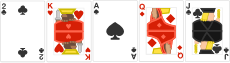### Data Structures

If there has been one recurring theme so far, it’s that more organized data can be valuable for certain types of computations, like searching for an element in a list. Specifically, we’ve noted that having a sorted list is generally more useful than having an unsorted list.

So, how can we sort a list? As with searching, we’ll start with a naive, intuitive approach (called insertion sort). In a later quiz, we’ll explore more efficient methods of sorting.

# Insertion Sort

Let $A$ be an array sorted in increasing order (from smallest to largest), and let $x$ be a single element outside of it. The natural question is, how can we place $x$ into our array while still keeping it sorted?

This operation is known as an insertion, and there are multiple ways to do it. However, in this chapter we will only concern ourselves with the method used in insertion sort, which uses the steps outlined below.

1. Place $x$ at the end of $A$.
2. Consider the element to the left of $x$. There are three possibilities.
• If there is no element to the left, then we are done, since $x$ will be the smallest element and it is already at the start of the array.
• If $x$ is greater than or equal to it, then we are done; $x$ is on the right of all smaller elements and on the left of all larger elements, so the array is once again sorted.
• If $x$ is smaller, then it should come before the other element. Switch the two to put $x$ in front.
3. Repeat step two until finished.

With each repetition of step two, we will either finish inserting, or reduce the number of elements ahead of $x$ by one. Since we are also finished when no more elements remain in front of $x$, repeating step two is guaranteed to successfully make an insertion.

# Insertion Sort

Suppose we have an array, [1, 3, 6, 7, 10], and a new element to add, $x = 4$. If we use the insertion procedure from our last slide to add $x$ to our array, how many comparisons will it take and what will be the final index of $x$ in the array?

Remember that arrays are indexed starting from zero, so the first element would correspond with index $0$, the second with index $1$, and so on.

Hint: Make sure you're following the algorithm we went over earlier. Remember that we make comparisons going from right to left.

# Insertion Sort

The full insertion sort algorithm works by dividing an array into two pieces, a sorted region on the left and an unsorted region on the right. Then, by repeatedly inserting elements from the unsorted half into the sorted half, the algorithm eventually produces a fully sorted array. The full steps of this process for an array, $A$, are shown below

• Designate the leftmost element of $A$ as the only element of the sorted side. This side is guaranteed to be sorted by default, since it now contains only one element.
• Insert the first element of the unsorted side into the correct place in the sorted side, increasing the number of sorted elements by one.
• Repeat step two until there are no unsorted elements left.

Notice that this method doesn’t require us to create a new array to store the sorted values. All we have to do is keep track of how much of the original array is sorted. This makes insertion sort an in-place algorithm.

# Insertion Sort

To summarize, Insertion sort is a sorting algorithm that builds a final sorted array one element at a time. The following is a visual representation of how insertion sort works:Insertion Sort:

• We start from the left. At first there is one element (which is, trivially, already sorted).

• Colloquially speaking, for each subsequent element, we compare it to each of the elements to the left of it, moving right to left, until we find where it “fits” in the sorted portion of the array.

• Since we are inserting the new element into the side of the array that is already sorted, the result will still be sorted.

• After we’ve inserted every element, we have a sorted array!

# Insertion Sort

Suppose you had the following poker hand dealt to you, and you decide to use insertion sort to sort it from smallest to largest:Note that $\mathbf{A>K>Q>J>2.}$ How many individual comparisons between cards would need to be made for the sort if we strictly stick to the instructions for insertion sort shown earlier?

Reminder: First, the $K$ and $2$ are compared. Then, the $A$ and $K$ are compared, then the $Q$ and $A$ and so on.

# Insertion Sort

Ari wants to prove that insertion sort is slow. What order should he put the cards in to make sorting the cards in ascending order maximally inefficient?

# Insertion Sort

In the worst case for a list with 10 distinct elements, how many comparisons between list elements are made?

# Insertion Sort

In the best case for a list with 10 distinct elements, how many comparisons are made?

# Insertion Sort

The worst case increases like $n^2$ for large $n,$ while the best case increases linearly (like $n$). Unfortunately for insertion sort—as we’ll explore in the next quiz—the worst case is much more likely, and the average case also increases like $n^2.$

Thankfully, we can do better. In later quizzes, we’ll dive into more complex, more efficient sorting algorithms; their worst case performance will increase like $n \log n$ for large $n.$

Before we get there, we need to take a detour to start formalizing this language of computational complexity. How do we actually express how many computations an algorithm will use, and how do we meaningfully compare algorithms? We’ll explore this in the next quiz, on big O notation.

# Insertion Sort

×